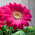## Implement Queue using Stacks

Implement the following operations of a queue using stacks.
• push(x) -- Push element x to the back of queue.
• pop() -- Removes the element from in front of queue.
• peek() -- Get the front element.
• empty() -- Return whether the queue is empty.
Notes:

• You must use only standard operations of a stack -- which means only push to toppeek/pop from topsize, and is empty operations are valid.
• Depending on your language, stack may not be supported natively. You may simulate a stack by using a list or deque (double-ended queue), as long as you use only standard operations of a stack.
• You may assume that all operations are valid (for example, no pop or peek operations will be called on an empty queue).

### Analysis:

This kind of problem usually requires more than one data structure to implement the other data structure. In this problem, two stacks are enough to implement a queue.

The idea is keep push element into stack 1, then "pop" is called, put all the elements from stack 1 to stack 2. Then pop the top element in stack 2.  If "pop" is called again, not stack 2 is not empty, just pop the top element is enough. If stack 2 is empty, put all the elements in stack 1 to stack 2.  In short, stack 1 is used for "push", stack 2 is used for "pop" and "peek". We do the "move element from stack1 to stack 2" only when stack 2 is empty and "pop" or "peek" is called.

e.g., We call push(1), push(2), push(3), push(4), and push(5), stack 1 is filled all the five elements, then do pop(), since stack 2 is empty, move elements from stack 1 to stack 2, then pop the top element (now is 1) in stack2:
Then we call push(6), push(7), push(8), push(9), and pop(), pop(), pop(), stack 1 is used for pushing, and stack 2 is used for popping :
Again, we call pop() (now 5 is popped out and no element in stack 2), and a peek() operation is called, stack 2 is empty, so push elements from stack 1 to stack 2, and return the top element as the peek:

### Code(C++):

class Queue {

stack<int> st1;
stack<int> st2;

public:
// Push element x to the back of queue.
void push(int x) {
st1.push(x);
}

// Removes the element from in front of queue.
void pop(void) {
if (!st2.empty()){
st2.pop();
}else{
while (!st1.empty()){
st2.push(st1.top());
st1.pop();
}
st2.pop();
}
}

// Get the front element.
int peek(void) {
if (!st2.empty()){
return st2.top();
}else{
while (!st1.empty()){
st2.push(st1.top());
st1.pop();
}
return st2.top();
}
}

// Return whether the queue is empty.
bool empty(void) {
return (st1.empty() && st2.empty());
}
};


### Code(Python):

class Queue(object):
def __init__(self):
"""
initialize your data structure here.
"""
self.st1 = []
self.st2 = []

def push(self, x):
"""
:type x: int
:rtype: nothing
"""
self.st1.append(x)

def pop(self):
"""
:rtype: nothing
"""
if len(self.st2) == 0:
while len(self.st1) != 0:
self.st2.append(self.st1.pop())
self.st2.pop()

def peek(self):
"""
:rtype: int
"""
if len(self.st2) == 0:
while len(self.st1) != 0:
self.st2.append(self.st1.pop())
return self.st2[-1]

def empty(self):
"""
:rtype: bool
"""
return not self.st1 and not self.st2


#### 1 comment:

1.